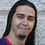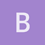Archive 04/01/2022.

[MrBayes] Weirid results in Tracer. Can someone help?tsmoreira

Hello PhyloPeople. I have a MrBayes issue here.

I`m trying to run a combined analysis (morphological + DNA) from my dataset, But I ran into some weird results and I do not know what to make of it…

I made four different datasets (with different number of taxa) trying to investigate effects of adding morphological data on the final topology. I have on the matrices in question, 4521 characters, 256 of those morphological. All datasets were analyze under the exact same parameters (Same data partitioning models and parameters) where the only variation was the taxa coverage:

• M1 has 307 taxa where 12 have only morphological data, 202 taxa have only molecular data and 93 have both
• M2 have 93 taxa where all of them have both morphological and molecular data
• M3 have 105 taxa where all of them have morphological data but only 93 have molecular data
• M4 have 294 taxa where all have molecular data, but only 93 have morphological data.

When I was checking my results of M3 (after 100 million generation) I got some very weird numbers on the ESS column on Tracer (See photo). At first I thought it was some parameterization problem… however I got the same issues on two of the four runs of M2 (the others seem ok.) M1 and M4 seem to be within the expected.

Did any of you guys had a similar issue before? What you think it my be the problem?

I appreciate any feedback you can give. Thanks!afmagee

It looks like there are 4 spikes, give or take. Since this is the combined analysis, that makes me suspicious that each analysis is in a very different region of parameter space (and thus when combining them the ESS is very bad).

What is each run doing? Are the ESS there also terrible? What is the ASDSF between the runs?

This isn’t a direct attack on the problem at hand, but identifying the mode of MCMC failure can help find the cause.afmagee

Wow… that really is bad (but helpful). The ASDSF is pretty clearly showing that runs are not near each other in treespace (the different TL and TH values also support this), which is bad but also not immediately an explanation for why the individual runs are doing so poorly. Extrapolating from those histograms, I would guess that the trees in each case are largely stuck in place.

It is interesting that this happens with the subsets with the most overlap. I wonder if the morphological and molecular datasets are at odds over the tree? Have you examined them separately? I wouldn’t think that 256 morphological characters would have the power to fight 4521 nucleotides over the tree topology, but perhaps they do (examining them separately would also let you see if they contribute enough to the likelihood to do that). How are you setting the “coding” option for the morphological characters? Are you allowing among-site rate variation for them?tsmoreira

It occurred me that. I tried to ran simplified analysis for shorter amount of generations (like 10KK) just to se how the chains behave. I also did tests isolating things like partition scheme, dating parameters…

The only test where the same phenomena occurred was I maintained the partition scheme (and excluded all other parameters sucha s clock rate, etc.)

Thats my MrBayes Block

outgroup Steatoda_grossa_THERIDIIDAE; constraint ingroup = 2-.;

set autoclose=no nowarn=yes;

charset Subset1 = 1-653; [16S] charset Subset2 = 654-2594; [18S] charset Subset3 = 2595-3883\3; [COI-1st] charset Subset4 = 2596-3883\3; [COI-2nd] charset Subset5 = 2597-3883\3; [COI-3rd] charset Subset6 = 3884-4265\3; [H3-1st] charset Subset7 = 3885-4265\3; [H3-2nd] charset Subset8 = 3886-4265\3; [H3-3rd] charset morpho = 4266-4521; [Morphology]

partition PartitionFinder = 9:Subset1, Subset2, Subset3, Subset4, Subset5, Subset6, Subset7, Subset8, morpho; set partition=PartitionFinder;

[GTR+I+G] lset applyto=(1) nst=6 rates=invgamma;

[SYM+I+G] lset applyto=(2) nst=6 rates=invgamma; prset applyto=(2) statefreqpr=fixed(equal);

[GTR+I+G] lset applyto=(3) nst=6 rates=invgamma;

[GTR+G] lset applyto=(4) nst=6 rates=gamma;

[GTR+I+G] lset applyto=(5) nst=6 rates=invgamma;

[SYM+I+G] lset applyto=(6) nst=6 rates=invgamma; prset applyto=(6) statefreqpr=fixed(equal);

[GTR+I+G] lset applyto=(7) nst=6 rates=invgamma;

[JC+I+G] lset applyto=(8) nst=1 rates=invgamma; prset applyto=(8) statefreqpr=fixed(equal);

[morpho] lset applyto=(9) coding=variable;

prset applyto=(all) ratepr=variable; unlink statefreq=(all) revmat=(all) shape=(all) pinvar=(all) tratio=(all);

prset nodeagepr = calibrated; prset brlenspr = clock:fossilization; prset speciationpr = exp(10); prset extinctionpr = beta(1,1); prset fossilizationpr = fixed(0); prset sampleprob = 0.01; prset samplestrat = diversity; prset topologypr = constraint(ingroup); prset treeagepr = unif(175,225);
prset clockratepr = lognorm(-1.90308998699,1);
prset clockvarpr = igr; prset igrvarpr = exp(10);

mcmcp ngen= 10000000 relburnin=yes burninfrac=0.33 printfreq=10000 samplefreq=10000 nchains=4 nruns=4 savebrlens=yes checkpoint=yes append=no;

mcmc; end;